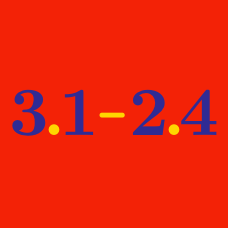Everyday Math

# Converting Decimals into Fractions

Which of the following is equal to $\frac{ 1}{2}$?

Which of the following is equal to $0.3$?

Which of the following is equal to $0.54$?

Express the following equation using fractions: $6.5 - 2.3 = 4.2.$

Which of the following is equal to $0.7$?

×